### Home > CALC > Chapter 6 > Lesson 6.3.2 > Problem6-93

6-93.
1. Find the following derivatives. Consider trigonometric substitutions to make your work easier. Homework Help ✎

1.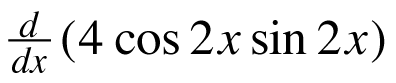2.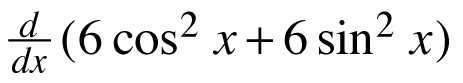3.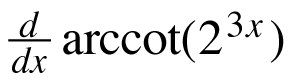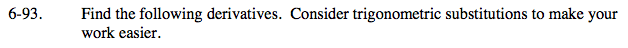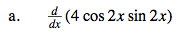Use a trigonometric identity AFTER you find the derivative.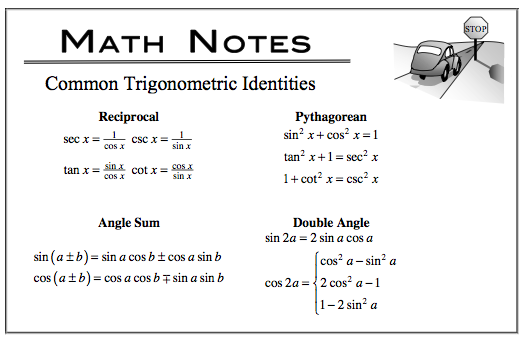4cos(2x)sin(2x) =
2sin(4x)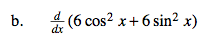Simplify the expression using a trigonometric identity BEFORE you take the derivative.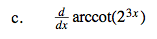Careful!

$\text{arccot}x\neq \frac{1}{\arctan x}$

$\text{arccot}x=\text{arctan}\left (\frac{1}{x} \right )$